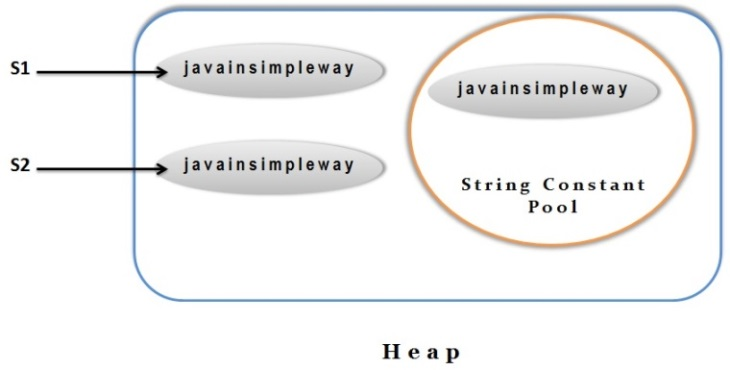# String comparison

#### There are many ways to do String comparison or equality check in Java

##### By using “==” operator

`==` operator always compares object’s references `not `the object content.

So, whenever we compare 2 strings using == operator it returns true only if they point to same memory location.

Even if they have same content but pointing to different memory locations, it returns false.

Example :

1. class StringCompare1{
2.  public static void main(String args[]){
3.
4.    String s1=new String("javainsimpleway");
5.    String s2=new String("javainsimpleway");
6.    System.out.println(s1==s2);
7.  }
8. }
```class StringCompare1{
public static void main(String args[]){

String s1=new String("javainsimpleway");
String s2=new String("javainsimpleway");
System.out.println(s1==s2);
}
}```

In the above program, we can see that s1 and s2 are pointing to different memory location and hence returning false

###### Same can be understood through below diagramConsider below example

1. class StringCompare2{
2.  public static void main(String args[]){
3.
4.    String s1= "javainsimpleway";
5.    String s2=”javainsimpleway";
6.   System.out.println(s1==s2);
7. }
8. }
```class StringCompare2{
public static void main(String args[]){

String s1= "javainsimpleway";
String s2=”javainsimpleway";
System.out.println(s1==s2);
}
}```

In the above program, we can see that s1 and s2 are referring to same memory location as we created them using string literal and hence only one string will be stored in string constant pool, Hence its returning true.

###### Same can be understood through below diagram###### Note :

Always remember that == compares 2 references not the actual content and hence its not safe comparison.

##### By using equals() method

This method compares 2 strings with their content character by character.

So, this method returns true if 2 strings have exactly `same `content and false otherwise.

Example :

1. class StringCompare3{
2.  public static void main(String args[]){
3.    String s1=new String("javainsimpleway");
4.    String s2=new String("javainsimpleway");
5.    System.out.println(s1.equals(s2));
6.  }
7. }
```class StringCompare3{
public static void main(String args[]){
String s1=new String("javainsimpleway");
String s2=new String("javainsimpleway");
System.out.println(s1.equals(s2));
}
}```

In the above program, we can see that s1 and s2 are pointing to different memory locations but equals() method compares the content of s1 and s2.

Since the content of both the strings is `same`, it returns true.

###### Note :

Always prefer using equals () method over == for comparing strings as we need to compare the actual content of the strings in most of our requirements.

##### By using equalsIgnoreCase() method

This method compares 2 strings with their content character by character by ignoring the case.

So, this method returns true if 2 strings have exactly `same `content with difference in character’s case and false otherwise.

So 2 strings having `same `content but differ in case where one being in upper case and other being lower case or mixed up of cases, this method consider them as `same `and returns true.

Example :

1. class StringCompare4{
2.  public static void main(String args[]){
3.    String s1=new String("JavaInSimpleWay");
4.    String s2=new String("javainsimpleway");
5.    System.out.println(s1.equals(s2));
6.    System.out.println(s1.equalsIgnoreCase(s2));
7.
8.  }
9. }
```class StringCompare4{
public static void main(String args[]){
String s1=new String("JavaInSimpleWay");
String s2=new String("javainsimpleway");
System.out.println(s1.equals(s2));
System.out.println(s1.equalsIgnoreCase(s2));

}
}```

In the above program, we can see that s1 and s2 are pointing to different memory locations but equals() method compares the content of s1 and s2.

Since the content of both the strings is `same`, but there is a difference in case where “J”,”I”,”S” and “W” are in upper case in s1 and in lower case in s2, equals() method returns false but equalsIgnoreCase() method returns true.

###### Note :

Prefer using equalsIgnoreCase() method for string comparison when we are not sure about other string is in upper case or lower case and case is not important in the requirement.

If data is case sensitive then use equals() method only

##### By using compareTo() method

This method also compares 2 strings but instead of returning Boolean output , it returns integer value which describes whether strings are equal or not.

If 2 strings are exactly same then this method returns `zero`.

If we compare 2 strings “s1” and “s2” then this method returns as below

S1 and s2 are same: `0`

S1 appears first compared to s2 in alphabetical order: `negative value`

S1 appears next compared to s2 in alphabetical order: `positive value`

Example :

1. class StringCompare5{
2.  public static void main(String args[]){
3.    String s1="java in simple way";
4.    String s2="java in simple way";
5.    String s3="java in easy way";
6.
7.    System.out.println(s1.compareTo(s2));//0 as s1 and s2 are same
8.    System.out.println(s1.compareTo(s3));//positive as  s1>s3
9.    System.out.println(s3.compareTo(s1));//negative as  s3 < s1
10.  }
11. }
```class StringCompare5{
public static void main(String args[]){
String s1="java in simple way";
String s2="java in simple way";
String s3="java in easy way";

System.out.println(s1.compareTo(s2));//0 as s1 and s2 are same
System.out.println(s1.compareTo(s3));//positive as  s1>s3
System.out.println(s3.compareTo(s1));//negative as  s3 < s1
}
}```

###### Note :

This method of string comparison is generally used in sorting logic as we need to identify whether strings are equal or lesser or greater to determine the order of elements in sorting.